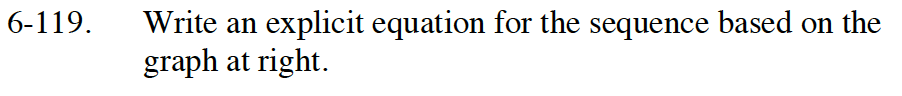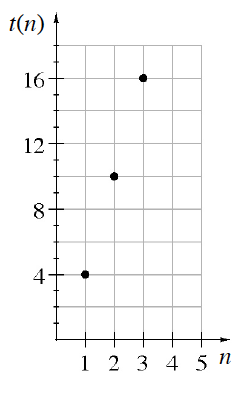Home > GB8I > Chapter cc46 > Lesson cc46.4.1 > Problem6-119

6-119.
1. Write an explicit equation for the sequence based on the graph at right. Homework Help ✎The x-axis represents the number of the term in the sequence.
The y-axis represents the value of the term. You can use the equation of the line that goes through
the points on the graph to find the relationship between the term and its value.

t(n) = 6n − 2